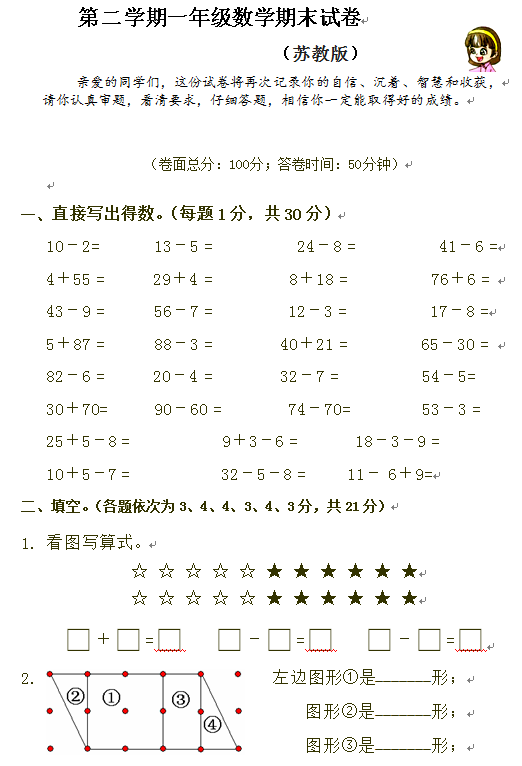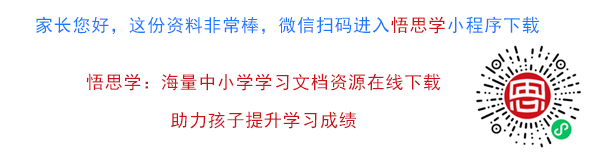苏教版

（卷面总分：100分；答卷时间：50分钟）

10－2=       13－5 =          24－8 =          41－6 =

4＋55 =    29＋4 =         8＋18 =          76＋6 =

43－9 =     56－7 =        12－3 =         17－8 =

【DOC文档4页】小学苏教版一年级下册数学期末试卷A4电子版资料_可直接打印_会员免费下载##### 评论信息